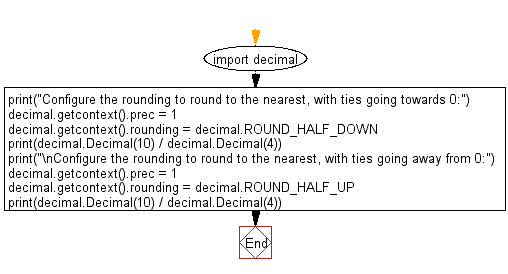﻿ Python: Configure the rounding to round to the nearest - with ties going towards 0, with ties going away from 0 - w3resource

# Python: Configure the rounding to round to the nearest - with ties going towards 0, with ties going away from 0

## Python module: Exercise-5 with Solution

Write a Python program that can be configured to round to the nearest - with ties going towards 0 and ties going away from 0. Use decimal.ROUND_HALF_DOWN, decimal.ROUND_HALF_UP

Sample Solution:

Python Code:

``````import decimal
print("Configure the rounding to round to the nearest, with ties going towards 0:")
decimal.getcontext().prec = 1
decimal.getcontext().rounding = decimal.ROUND_HALF_DOWN
print(decimal.Decimal(10) / decimal.Decimal(4))
print("\nConfigure the rounding to round to the nearest, with ties going away from 0:")
decimal.getcontext().prec = 1
decimal.getcontext().rounding = decimal.ROUND_HALF_UP
print(decimal.Decimal(10) / decimal.Decimal(4))
```
```

Sample Output:

```Configure the rounding to round to the nearest, with ties going towards 0:
2

Configure the rounding to round to the nearest, with ties going away from 0:
3
```

Flowchart:## Visualize Python code execution:

The following tool visualize what the computer is doing step-by-step as it executes the said program:

Python Code Editor:

Have another way to solve this solution? Contribute your code (and comments) through Disqus.

What is the difficulty level of this exercise?

Test your Programming skills with w3resource's quiz.

﻿

## Python: Tips of the Day

Inverts a dictionary with non-unique hashable values:

Example:

```def tips_collect_dictionary(obj):
inv_obj = {}
for key, value in obj.items():
inv_obj.setdefault(value, list()).append(key)
return inv_obj
ages = {
"Owen": 25,
"Jhon": 25,
"Pepe": 15,
}
print(tips_collect_dictionary(ages))
```

Output:

```{25: ['Owen', 'Jhon'], 15: ['Pepe']}
```

We are closing our Disqus commenting system for some maintenanace issues. You may write to us at reach[at]yahoo[dot]com or visit us at Facebook